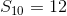# AP Calculus BC : Geometric Series

## Example Questions

### Example Question #1 : Series Of Constants

Consider: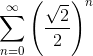.   Will the series converge or diverge? If converges, where does this coverge to?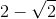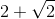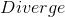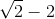Explanation:

This is a geometric series.  Use the following formula, whereis the first term of the series, andis the ratio that must be less than 1.  Ifis greater than 1, the series diverges.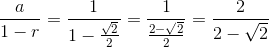Rationalize the denominator.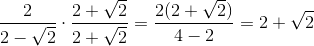### Example Question #2 : Series Of Constants

Consider the following summation: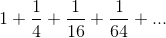.  Does this converge or diverge?  If it converges, where does it approach?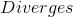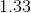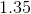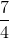Explanation:

The problem can be reconverted using a summation symbol, and it can be seen that this is geometric.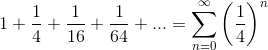Since the ratio is less than 1, this series will converge.  The formula for geometric series is: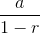whereis the first term, andis the common ratio.  Substitute these values and solve.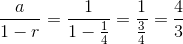### Example Question #3 : Series Of Constants

A worm crawls up a wall during the day and slides down slowly during the night. The first day the worm crawls one meter up the wall. The first night the worm slides down a third of a meter. The second day the worm regains one third of the lost progress and slides down one third of that distance regained on the second night. This pattern of motion continues...

Which of the following is a geometric sum representing the distance the worm has travelled after12-hour periods of motion? (Assuming day and night are both 12 hour periods).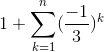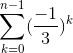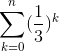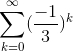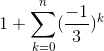Explanation:

The sum must be alternating, and after one period you should have the worm at 1m. After two periods, the worm should be at 2/3m. There is only one sum for which that is true.

### Example Question #7 : Concepts Of Convergence And Divergence

Determine whether the following series converges or diverges. If it converges, what does it converge to?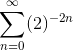Explanation:

First, we reduce the series into a simpler form.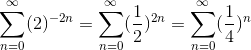We know this series converges because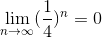By the Geometric Series Theorem, the sum of this series is given by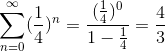### Example Question #4 : Series Of Constants

Calculate the sum of a geometric series with the following values: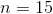,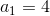,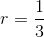. Round the answer to the nearest integer.Explanation:

This is a geometric series.

The sum of a geometric series can be calculated with the following formula,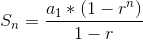, where n is the number of terms to sum up, r is the common ratio, and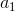is the value of the first term.

For this question, we are given all of the information we need.

Solution: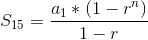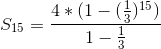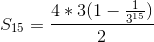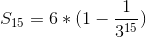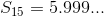Rounding,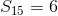### Example Question #5 : Series Of Constants

Calculate the sum, rounded to the nearest integer, of the first 16 terms of the following geometric series: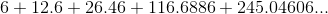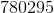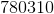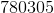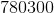Explanation:

This is a geometric series.

The sum of a geometric series can be calculated with the following formula,, where n is the number of terms to sum up, r is the common ratio, andis the value of the first term.

We haveand n and we just need to find r before calculating the sum.

Solution: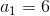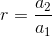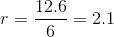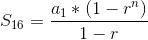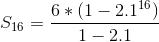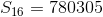### Example Question #6 : Series Of Constants

Calculate the sum of a geometric series with the following values: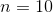,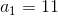,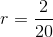,

rounded to the nearest integer.Explanation:

This is a geometric series.

The sum of a geometric series can be calculated with the following formula,, where n is the number of terms to sum up, r is the common ratio, andis the value of the first term.

For this question, we are given all of the information we need.

Solution: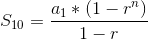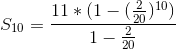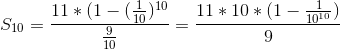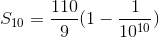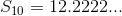Rounding,